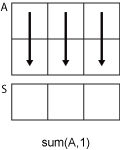# sum

## 语法

``S = sum(A)``
``S = sum(A,'all')``
``S = sum(A,dim)``
``S = sum(A,vecdim)``
``S = sum(___,outtype)``
``S = sum(___,nanflag)``

## 说明

``S = sum(A)` 返回 A 沿大小不等于 1 的第一个数组维度的元素之和。如果 `A` 是向量，则 `sum(A)` 返回元素之和。如果 `A` 是矩阵，则 `sum(A)` 将返回包含每列总和的行向量。如果 `A` 是多维数组，则 `sum(A)` 沿大小不等于 1 的第一个数组维度计算，并将这些元素视为向量。此维度会变为 `1`，而所有其他维度的大小保持不变。`

``S = sum(A,'all')` 计算 `A` 的所有元素的总和。此语法适用于 MATLAB® R2018b 及更高版本。`

``S = sum(A,dim)` 沿维度 `dim` 返回总和。例如，如果 `A` 为矩阵，则 `sum(A,2)` 是包含每一行总和的列向量。`

``S = sum(A,vecdim)` 根据向量 `vecdim` 中指定的维度对 `A` 的元素求和。例如，如果 `A` 是矩阵，则 `sum(A,[1 2])` 是 `A` 中所有元素的总和，因为矩阵的每个元素包含在由维度 1 和 2 定义的数组切片中。`

``S = sum(___,outtype)` 使用上述语法中的任何输入参数返回指定数据类型的总和。`outtype` 可以是 `'default'`、`'double'` 或 `'native'`。`

``S = sum(___,nanflag)` 指定在上述任意语法的计算中包括还是忽略 `NaN` 值。`sum(A,'includenan')` 会在计算中包括所有 `NaN` 值，而 `sum(A,'omitnan')` 则忽略这些值。`

## 示例

```A = 1:10; S = sum(A)```
```S = 55 ```

`A = [1 3 2; 4 2 5; 6 1 4]`
```A = 3×3 1 3 2 4 2 5 6 1 4 ```
`S = sum(A)`
```S = 1×3 11 6 11 ```

`A = [1 3 2; 4 2 5; 6 1 4]`
```A = 3×3 1 3 2 4 2 5 6 1 4 ```
`S = sum(A,2)`
```S = 3×1 6 11 11 ```

`A = ones(4,3,2);`

`S1 = sum(A,[1 2])`
```S1 = S1(:,:,1) = 12 S1(:,:,2) = 12 ```

`S2 = sum(A,[2 3])`
```S2 = 4×1 6 6 6 6 ```

`S3 = sum(A,[1 3])`
```S3 = 1×3 8 8 8 ```

`S4 = sum(A,[1 2 3])`
```S4 = 24 ```
`Sall = sum(A,'all')`
```Sall = 24 ```

```A = ones(4,2,3); S = sum(A,3)```
```S = 4×2 3 3 3 3 3 3 3 3 ```

```A = int32(1:10); S = sum(A,'native')```
```S = int32 55 ```

```A = [1.77 -0.005 3.98 -2.95 NaN 0.34 NaN 0.19]; S = sum(A,'omitnan')```
```S = 3.3250 ```

## 输入参数

• 如果 `A` 是标量，则 `sum(A)` 返回 `A`

• 如果 `A` 是 0×0 空矩阵，则 `sum(A)` 返回 `0`

• `sum(A,1)``A` 的列中的连续元素进行求和并返回一个包含每列之和的行向量。• `sum(A,2)``A` 的行中的连续元素进行求和并返回一个包含每行之和的列向量。`dim` 大于 `ndims(A)` 或者 `size(A,dim)``1` 时，`sum` 返回 `A``outtype`输出数据类型
`'default'``double`，但当输入数据类型为 `single``duration` 时，输出类型则为 `'native'`
`'double'``double`，但当数据类型为 `duration` 时不支持 `'double'` 类型
`'native'`与输入相同的数据类型，但当输入数据类型为 `char` 时不支持 `'native'`

`NaN` 条件，指定为下列值之一：

• `'includenan'` - 计算总和时包括 `NaN` 值，生成 `NaN`

• `'omitnan'` - 忽略输入中的所有 `NaN` 值。